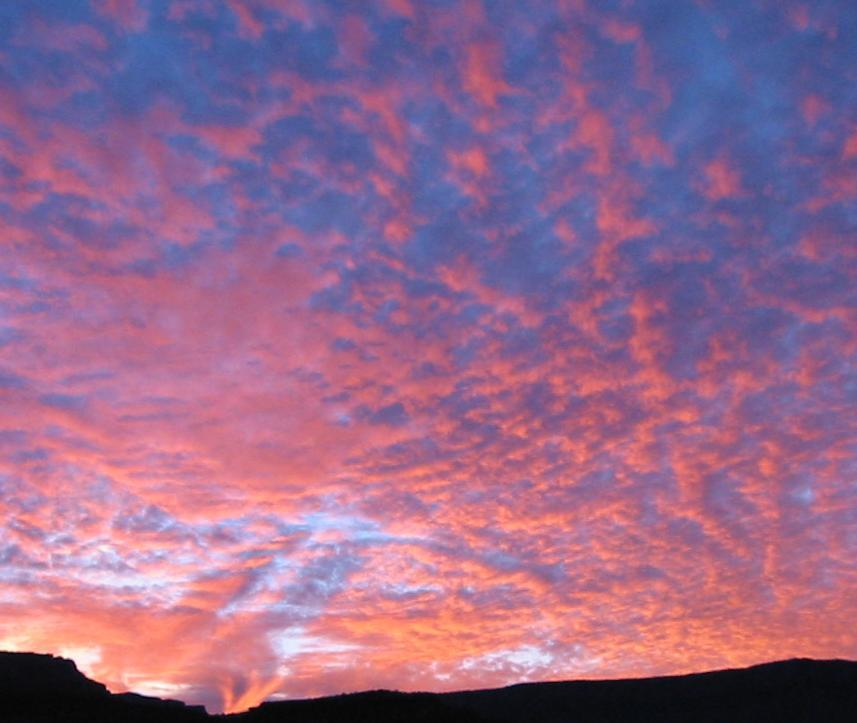# Atoms

Find the elements that compose the atomsE
G
I
R
N
S
U
T
V
X
K
T
R
A
A
T
L
N
C
L
O
Y
Q
C
G
A
N
Q
T
T
N
Z
E
K
U
E
I
T
S
B
Q
E
L
V
B
X
O
F
C
T
K
L
S
G
X
L
G
V
P
R
D
T
R
U
T
R
H
F
S
Y
I
A
H
I
O
S
K
D
T
F
R
L
H
H
I
Y
T
D
J
E
B
K
H
B
U
W
O
Y
C
T
F
I
B
O
V
V
K
M
A
S
E
B
N
V
F
V
V
W
W
N
N
F
E
J
I
B
N
E
I
E
I
E
U
E
Q
P
D
R
S
O
S
V
B
S
J
P
B
R
A
F
R
C
D
X
N
S
G
E
V
I
T
I
S
O
P
O
V
C
J
Z
M
J
K
L
N
W
Q
L
G
H
T
K
H
A
A
W
U
C
B
R
P
G
Q
F
U
O
F
T
Z
B
W
O
O
V
L
C
Y
V
G
D
N
D
B
U
G
O
T
Y
U
X
Z
A
V
O
M
Show words that are in the puzzle

### Words

1. What is the particle that is always floating aroung the atom?
2. What is the neutral particle of an atom
3. What is the atom that is in the nucleus, together with the Neutron?
4. What is the charge of the electrons?
5. What is the charge of the protons?
6. When we break the nucleus of an atom, energy is produced by...
7. When we join two nucleai of different atoms, the energy is produced by...

#### Quick Feedback for Knowledge Mouse

Want to suggest a feature? Report a problem? Suggest a correction? Please let Knowledge Mouse know below: